## General Question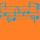# Is 3 ever a divisor or 2^n?

Asked by dxs (15085) January 12th, 2016

Note: In an effort to avoid text formatting issues, I’m using two carets to denote exponentiation. So, x^^n means x to the nth power. I hope this works.

2^^n/3. Is it ever an integer?
Intuitively, it seems not. Is it really simple to (dis)prove it and I’m thinking too hard? I tried algebra/logarithm stuff, and had no luck.

The next step I took was to make some sort of function mapping the powers of 2 to the distance between the powers of 2. That is, I wanted to show that the distance between the powers is a quantity that is a power of 2. (Ex: the distance between 2^^6 and 2^^5 is 32, which equals 2^^5. This means I’m mapping the input power to the power after it (2^^n maps to a function of 2^^(n+1) [f(2^^n)=2^^(n+1)/2 to be exact]). Showing that they’re tied to each other in this way, I thought it could prove that these numbers will never be in 3ℤ by showing the mapping goes on forever. Not sure this will get me there, though.

I was thinking of further generalizing it to any co-prime pair of integers. (gcd(n,m)=1 ⇒ m∤n^^p). Actually, you can go further. gcd(14,35) is 7, and no power of either will ever (seemingly) divide the other. 35∤14^^p & 14∤35^^p.

Observing members: 0Composing members: 0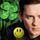Think of the basic rules of automata.
Prove the starting point., n=1 -> ⅔, Nope.
Now prove the next step n=n+1 2×⅔ Nope.
Now increment by proving all successive steps are a multiple of one of the above steps.

(Unless I misunderstood the question.)

LuckyGuy (38449)“Great Answer” (1) Flag as…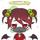for every number that is divisible by 3?
like: 3, 6, 9, 12, 15, 18, 21, etc.?
2^3/3=2
2^6/3=4
2^9/3=8
etc.

ragingloli (47256)“Great Answer” (2) Flag as…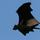No, of course not. All of the powers of 2 have that digit as their only (basic) divisor. There is no power of 2 value that is also a multiple of 3.

2^2 = 2×2 = 4, not divisible by 3
2^3 = 2×2 x 2 = 8, ditto
2^4 = 2×2 x 2×2 =16, ditto
2^5 = 2×2 x 2×2 x 2 = 32, ditto
...
2^x = 2×2 x 2×2 x…. X 2 (“x” times). There is no 3 in that succession. So there is no way to divide any product of 2 evenly by 3.

CWOTUS (26082)“Great Answer” (3) Flag as…@ragingloli @LuckyGuy I mean to say (2^n)/3. The carets make things whispery when I use only one, so the notation became really confusing.
@LuckyGuy I tried induction as well. It lead me nowhere since it just showed what I was trying to prove.

Aside: This thought came about when someone told me that they were ⅓ Italian. I’m not sure if he was joking, but I laughed.

dxs (15085)“Great Answer” (1) Flag as…In the interests of accuracy, I should have concluded “no way to divide any power of 2 evenly by 3.”

@CWOTUS regrets the error.

CWOTUS (26082)“Great Answer” (0) Flag as…You know, I probably should’ve had a preposition in the original question instead of a conjunction, but I’ll be okay if this gets modded off. My problem has been solved.

dxs (15085)“Great Answer” (0) Flag as…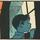@CWOTUS Has the right approach. One can make a generalization that any x^n where x is prime is not divisible by any other prime number because only x is a prime factor.

zenvelo (35432)“Great Answer” (5) Flag as…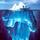There’s something known as the Fundamental Theorem of Arithmetic (aka Unique Factorization Theorem) (Wiki) that says every integer greater than 1 is either prime or is a product of primes, which product is unique up to the order of the factors. In other words, there’s only one way to decompose a number into primes. So if N = 2^n for some integer n, then N has only factors of 2—it cannot alternatively be decomposed into primes some other way. So 3 cannot be a factor of a power of 2.

The theorem isn’t all that obvious, so it’s a reasonable question, especially when you get into very large numbers. I’ve seen the proof in a textbook on number theory & it’s not so simple.

gasman (11313)“Great Answer” (1) Flag as…The proof is not difficult, but it has one tricky part.
Here is an outline.

1. First you have to understand Euclid’s algorithm for finding the gcd(a,b). This is not commonly taught, but it is a simple and pretty result, which is based on the following easily proved result: If a > b then gcd(a,b) = gcd(a – b, b). This reduces the original gcd problem to one using smaller numbers and you keep applying the rule until you reach the point where a’ = b’, which will be gcd(a,b).

2. Next, realize that by keeping track of what you did in the first step, you can get two numbers x and y such that ax + by = gcd(a,b)

3. Here is the tricky part. We are going to use result 2 to prove the following: if a divides bc and a and b are relatively prime then a divides c. Relatively prime means that a and b have no common factor other than 1, so gcd(a,b) = 1, and by (2) that means that there are numbers m and n such that am + bn = 1. (Here comes the trick) Multiply both sides by c. Then:
amc + bcn = c. a divides amc (obviously) and a divides bcn, because we are given that it divides bc. Then a must divide the righthand side, so a divides c.

4. It is easy to show that every number has some prime factorization. If a number is not itself prime then it is the product of two smaller numbers, and then we can show by induction that each of the two smaller numbers has some prime factorization.

5. The hard part is showing that any two prime factorizations are the same. If n = p1p2p3…pn = q1q2…qm, consider p1. If p1 is different from q1 then they must be relatively prime and by (3) p1 must divide q2q3…qm. We continue in this way, showing that p1 must divide one of the qi. The same holds for all the pi, so the factorizations must be the same.

LostInParadise (27917)“Great Answer” (1) Flag as…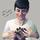The prime factorization of 2^n, which reveals all the prime numbers it is divisible by, will contain only twos, so no.

Mariah (25863)“Great Answer” (2) Flag as…or